# 机器学习| K-近邻算法详解

### 最近邻算法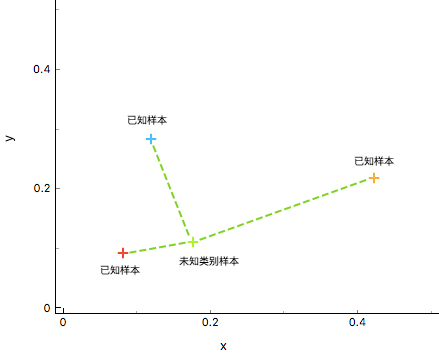### K-近邻算法

K-近邻（K-Nearest Neighbors，简称：KNN）算法是最近邻（NN）算法的一个推广，也是机器学习分类算法中最简单的方法之一。KNN 算法的核心思想和最近邻算法思想相似，都是通过寻找和未知样本相似的类别进行分类。但 NN 算法中只依赖 1 个样本进行决策，在分类时过于绝对，会造成分类效果差的情况，为解决 NN 算法的缺陷，KNN 算法采用 K 个相邻样本的方式共同决策未知样本的类别,这样在决策中容错率相对于 NN 算法就要高很多，分类效果也会更好。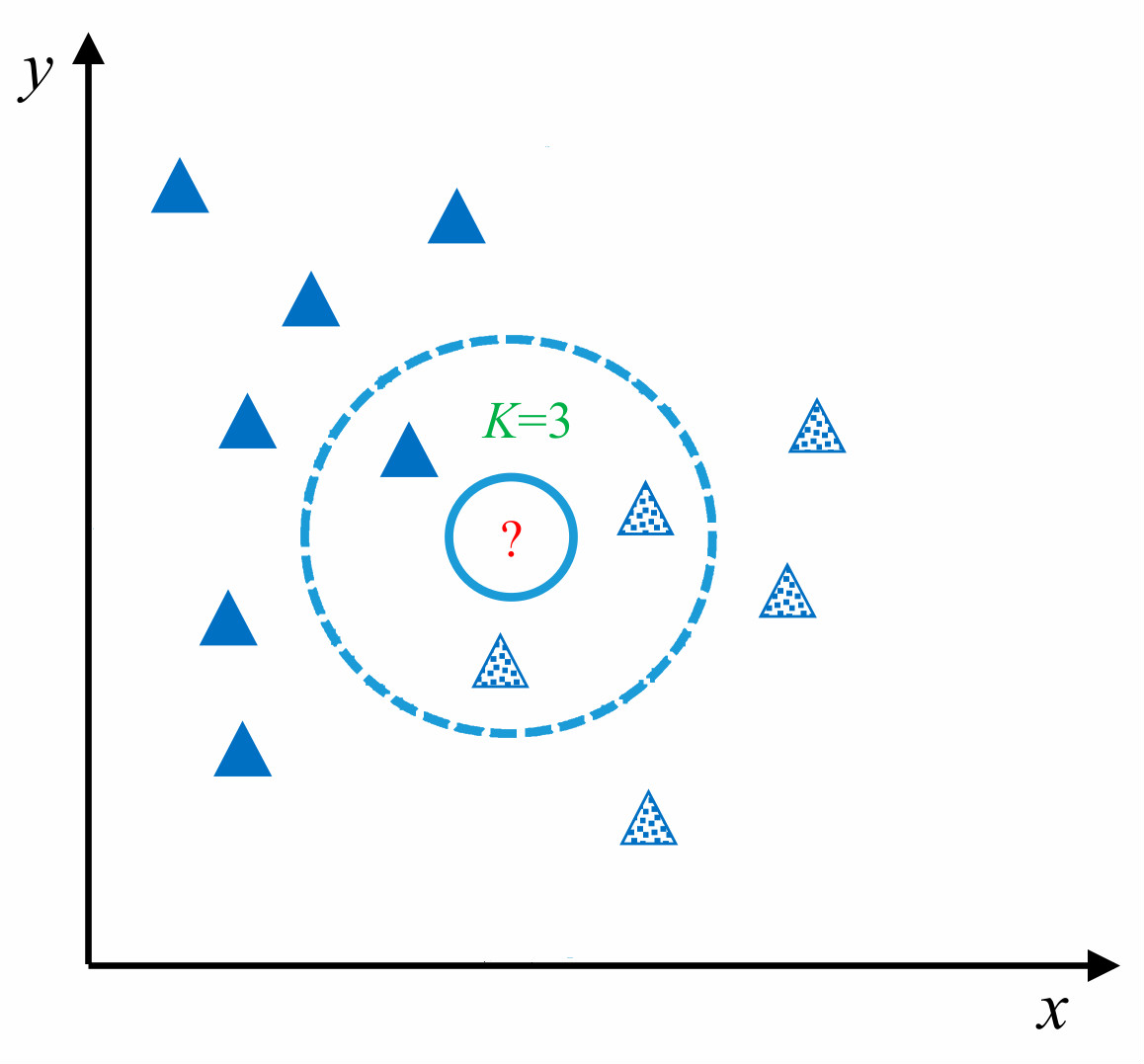## K-近邻算法实现

KNN 算法在理论上已经非常成熟，其简单、易于理解的思想以及良好的分类准确度使得 KNN 算法应用非常广泛。算法的具体流程主要是以下的 4 个步骤：

1. 数据准备：通过数据清洗，数据处理，将每条数据整理成向量。
2. 计算距离：计算测试数据与训练数据之间的距离。
3. 寻找邻居：找到与测试数据距离最近的 K 个训练数据样本。
4. 决策分类：根据决策规则，从 K 个邻居得到测试数据的类别。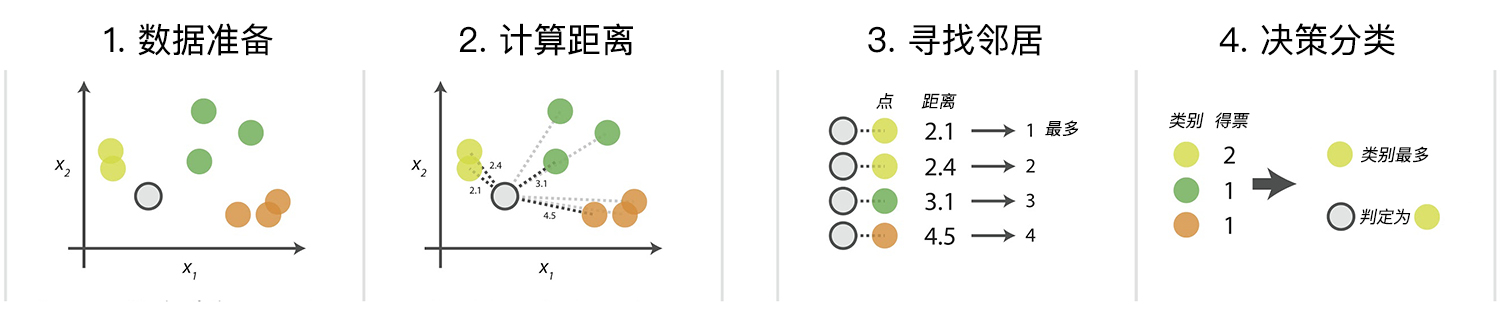### 数据生成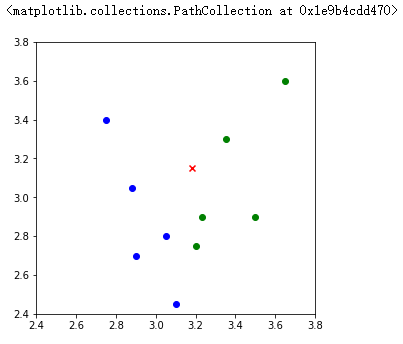### 距离度量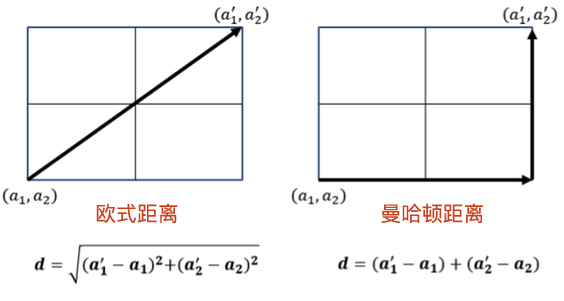#### 曼哈顿距离

$$d_{man}=\sum_{i=1}^{N}\left | X_{i}-Y_{i} \right |$$

• $X$,$Y$：两个数据点
• $N$：每个数据中有 $N$ 个特征值
• $X_{i}$ ：数据 $X$ 的第 $i$ 个特征值

#### 欧式距离

$$d_{euc}= \sqrt{\sum_{i=1}^{N}(X_{i}-Y_{i})^{2}}$$

• $X$, $Y$ ：两个数据点
• $N$：每个数据中有 $N$ 个特征值
• $X_{i}$ ：数据 $X$ 的第 $i$ 个特征值

### 决策规则

• 多数表决法：多数表决法类似于投票的过程，也就是在 K 个邻居中选择类别最多的种类作为测试样本的类别。
• 加权表决法：根据距离的远近，对近邻的投票进行加权，距离越近则权重越大，通过权重计算结果最大值的类为测试样本的类别。

### KNN 算法实现

• test_data：用于分类的输入向量。
• train_data：输入的训练样本集。
• labels：样本数据的类标签向量。
• k：用于选择最近邻居的数目。

### 可视化展示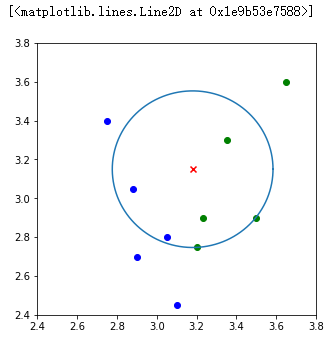laugh12321

2019-01-07

2020-10-24